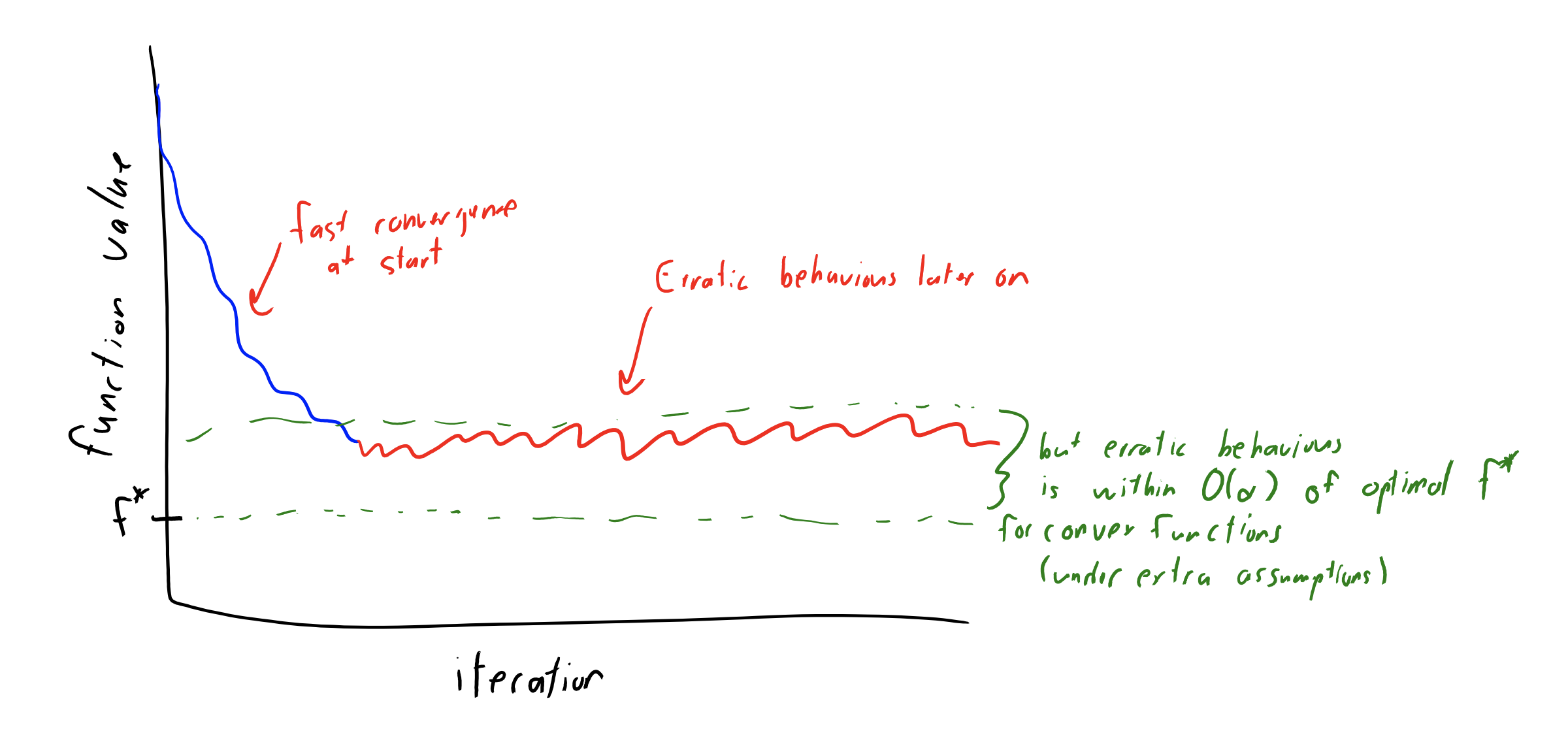When we minimize or maximize a function, we call it optimization.

Gradient descent is essentially an iterative optimization algorithm that takes a guess and refines it using the gradient to make a better guess.

If the objective function is a convex function, then it will converge to a global optimum.

Gradient descent finds critical point of differentiable function. Which can be faster than normal equations for large ‘d’ values. It takes per iteration so for iterations.

Formally,

2. We repeatedly refine the guess:
1. here is the learning rate.
2. We move in the negative gradient direction as given some parameters the direction of largest decrease is
3. We stop when

## Stochastic Gradient Descent (SGD) §

However, the runtime of each iteration of regular gradient descent is proportional to . This is problematic when we have large training sets!

Instead of computing the gradient over all training examples, we do it for some random training example . Intuition is that on average, the algorithm will head in the right direction

We can use it when minimizing averages (so all regression losses except brittle regression)

When we get close enough to a local minima , we enter a region of confusion where some point towards and others don’t. This confusion region is captured by the variance of the gradients

• If the variance is 0, every step goes in the right direction (outside region of confusion)
• If the variance is small, most steps go in the right direction (just inside region of confusion)
• If the variance is large, many steps point in the wrong direction (middle of region of confusion)

Basically, for a fixed stepsize, SGD makes progress until the variance is too large.### Decreasing Step Size §

If we decrease the step size as we keep training, we can still converge to a stationary point as long as:

A common option is to use

### Minibatches §

We can train on a ‘mini-batch’ of examples. Radius of region of confusion is inversely proportional to

### Early Stopping §

Normally, we stop GD when gradient is close to zero. However, we never know this when doing SGD (as we cannot see the full gradient). We just stop early if the validation set error is not improving (this also reduces overfitting)